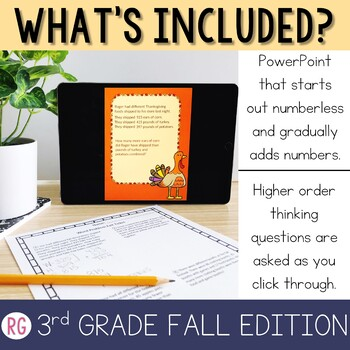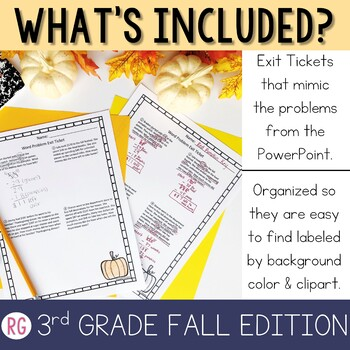Numberless Word Problems for 3rd Grade Fall Themed3rd - 4th
Subjects
Standards
Resource Type
Formats Included
• Zip
• Multimedia
Pages
68 pages

Also included in

1. Third Grade Numberless Addition, Subtraction, Multiplication, and Division Word Problems with Exit Tickets and Answer KeysDo you have a student that just starts to add, subtract, multiply, or divide a problem without ever really figuring out what is happening in the story problem? If so....ummm we a
\$23.00
\$32.50
Save \$9.50
2. Third Grade Numberless Addition, Subtraction, Multiplication, and Division Word Problems with Exit Tickets and Answer Keys for Fall,Spring,Winter and Summer. Do you have a student that just starts to add, subtract, multiply, or divide a problem without ever really figuring out what is happening in t
\$19.20
\$24.00
Save \$4.80

Description

These Numberless Word Problems can be used for your Digital Distance Learning in 3rd Grade. My blog post tells you how simple it can be.

These Fall Numberless Addition, Subtraction, Multiplication, and Division Word Problems included Numberless Word Problems and Exit Tickets with Answer Keys

Do you have a student that just starts to add, subtract, multiply, or divide a problem without ever really figuring out what is happening in the story problem? If so....ummm we all do.... then this resource is for you.

I ABSOLUTELY LOVE THIS RESOURCE AND USE IT WITH MY 3rd GRADERS EVERY DAY!

This is a resource that is created to help you teach word problem solving strategies.

When the "act it out" strategy doesn't work for your student this is a phenomenal strategy to try.

This strategy works because students can't just add or subtract the numbers before they ever actually read the problem.

This product guides them through the thinking and reasoning as to why they are adding or why they are subtracting. If students learn reasoning skills this will help them with many subjects in school as well as in life in general (the real-world).

INCLUDED:

*20 No Prep (Digital Slideshow) Addition, Subtraction, Multiplication and Division 1 and 2 step word problems.

• These problems start out numberless and then gradually show you the numbers and ask you to solve a problem.
• As you click through the interactive slideshow it provides you with prompting questions to help your students build reasoning behind the word problems.

*20 Exit tickets that follow the same process as the questions on each slide.

• These are helpful to use after each discussion to see if it is helping your students understand each type of problem.
• Exit Tickets are organized in the file for easy accessibility. The exit ticket titled "Purple Scarecrow" will go with the numberless problem with a purple background and scarecrow clip art, so you can easily print whichever exit ticket you need.
• Total of 75 more word problems for practice.

*Answer Keys for Exit tickets and Numberless word problems as well.

• Answer Keys on exit tickets are detailed to show the CUBES strategy and different processes and strategies students could use to solve the problems.

I have provided a list of sentence stems to help you guide the students to think in the correct direction, but there are already questions that come up on each slide so this these sentence stems are just extra if you want them.

USES:

This can be used whole group, small group, tutoring, or during intervention.

Covers

TEKS

3.4 A, 3.4 B, 3.4D, 3.4F, 3.4G, 3.4K

CC

3.NBT.2 , 3.OA.C.7, 3.OA.D.8, 4.OA.A.3

ALL QUESTIONS ARE TEST PREP WORTHY!

You might also like:

Numberless 3rd Winter Edition

Numberless 3rd Spring Edition

Numberless 3rd Bundle

Are you wondering how the Numberless 3rd Bundle is different than Numberless 3rd "Fall Edition" or "Winter Edition" ??

• These are different than this product because they do not have the "prompting questions" on each slide so this allows you to lead the discussion however you see fit.
• These also do not have exit tickets for each question but they include 30 questions instead of 20.
• These are also not "themed" aka "Fall".
Total Pages
68 pages
Included
Teaching Duration
2 Weeks
Report this Resource to TpT
Reported resources will be reviewed by our team. Report this resource to let us know if this resource violates TpT’s content guidelines.

Standards

to see state-specific standards (only available in the US).
Solve multistep word problems posed with whole numbers and having whole-number answers using the four operations, including problems in which remainders must be interpreted. Represent these problems using equations with a letter standing for the unknown quantity. Assess the reasonableness of answers using mental computation and estimation strategies including rounding.
Solve two-step word problems using the four operations. Represent these problems using equations with a letter standing for the unknown quantity. Assess the reasonableness of answers using mental computation and estimation strategies including rounding.
Fluently multiply and divide within 100, using strategies such as the relationship between multiplication and division (e.g., knowing that 8 × 5 = 40, one knows 40 ÷ 5 = 8) or properties of operations. By the end of Grade 3, know from memory all products of two one-digit numbers.
Fluently add and subtract within 1000 using strategies and algorithms based on place value, properties of operations, and/or the relationship between addition and subtraction.